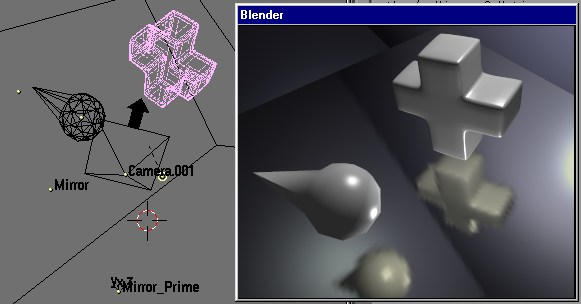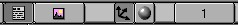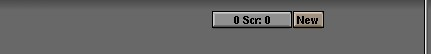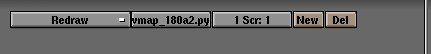Blender Python and EnvMap  To bind a script python to determine automatically  the position relative of the camera to a plan.
 Index Beginning Python:trucs precedent and easy ways 2 In Following building site

When we have a mirror in a scene, we are very annoyed each time the camera is moved  (or if we move the plan of the mirror itself)  because point by which the texture is calculated must be rebuilt too. And this texture can't  be used in an animation.It is not especially difficult if we use a plan parallel with a plan of the trihedron, that becomes unpleasing when this plan was rotated on three various angles  (see the method suggested in these pages .)

It would not be marvellous that Blender automatically readjusts this texture according to the modifications of positionnemement and orientations of empty which controls the texture? Alas that is not yet possible, but we can write a script python which will do it in its place.

The objective of this tutoriel is to have script and how to make use of it Then to detail different the elements necessary to the realization and the correct operation from this script, i.e.:
-- to calculate symmetrical orthogonal camera compared to the plan-mirror.
-- to position the nodes of the plan in an absolute way in space of Blender and to find truths coefficients
-- to link script to two objects to obtain the automatic repositioning of the empty on which the effect of reflexion is calculated.
What you must know to approach this tutoriel:
-- to recover a copy of a blender object through the API python
-- to know the structure of this object to get the good parameters
What is not this tutoriel:
-- an explanation of the texture of EnvMap, to see here for some explanations and details

Script
Two scripts are currently available: for the versions of Blender lower than the version 2.04, and another for version 2.12.
To charge the files:

EnvMap_O_Matic2_12 for the API python Blender 2.12
EnvMap_O_Matic1_80 for the API python Blender 1.80

The explanations which follow take the version 1.80a in example because it is the surest version and that which holds less surprises when with the nondesired results. Indeed the verison 2.12 functions but adds additional nodes in the mesh Plan. Experiment to try to include/understand the problem: edition of the plan touches, selection of all the nodes with the key has and their displacement with the key G What has the long one can be génant even dangeureux for the stability of the software.

Script is autonomous and functions without any additional importation, even not the module math If one wishes to adapt it to a personal realization it is enough to control the three principal objects which are:
 ..  PlaneObname=' Mirror'  EmptyObCibleName=' Mirror_Prime'  CameraName=' Camera.001 '

The ' Mirror' is simply the plan on which all must be reflected.
The ' Caméra.001 ' is the current camera.
The ' Mirror_Prime' is the object which is in the Ob button: the declaration of texture:Symmetrical orthogonal compared to a plan

It is the point which is contrary to the plan on an axis perpendicular to this plan and at a distance identical to that which separates the plan from the camera. In script, it is defined by the EmptyObCibleName variable The variable which indicates the name of the mirror plan is PlaneObName The camera is quite simply CameraName These variables must be documented with the names of the objects corresponding in the scene under development. If one of them is not correctly identified then script does not function and returns an error in the console.

 (this part of the tutoriel was provided by Didier Laffont, currently tahitien of adoption)  A plan of Cartesian equation u*x+v*y+w*z+h=0 with four is P constant U, v, W, H (U, v, W different from (0.0,0.0,0.0) and M° (x°, y°, z°) a point apart from the plan.  Then the co-ordinates (x°', y°', z°') of the orthogonal point M°' symmetrical of M° compared to the plan P should be except computational error (calculations which are saved to the reader):  x° ' = (-2(u*x°+v*y°+w*z°+h) * U) / (u²+v²+w²) + x°  y° ' = (-2(u*x°+v*y°+w*z°+h) * v) / (u²+v²+w²) + y°  z° ' = (-2(u*x°+v*y°+w*z°+h) * W) / (u²+v²+w²) + z°  From where  x° ' = A*u + x°  y° ' = A*v + y° with A=(-2(u*x°+v*y°+w*z°+h) * W) / (u²+v²+w²)  z° ' = A*w + z°  (Fine of the fundamental contribution of Didier Laffont, without which this script would not function.)

In the script which is proposed in accompaniment these results were transformed into a very short function:
 def point_miroite(nx, ny, nz, K, p):  With = -2*(nx*p+ny*p+nz*p+k)/(nx*nx+ny*ny+nz*nz)  return [ nx*A+p[0 ], ny*A+p[1 ], nz*A+p[2 ] ]

and U, v, W and H were replaced by nx, ny, nz and K

To find the coefficients of the nx

plan, ny, nz would correspond to the normal vector of the plan, knowing that, it would be enough to substitute any point of the plan to obtain K In fact the API Python of blender makes it possible to recover the normal vector associated with any node. But that would not be used for nothing because the vector in question would be calculated in the local reference mark of the plan itself and not the reference mark of the total space of blender where the camera is located.
For of this problem it is initially necessary to transpose the nodes of the plan in the total space of blender before using them to calculate truths coefficients. For that it is necessary to make interbvenir three transformations which are the localization, rotation and the scaling. One can know them by using key N The API python 1.80a offers the Object module to have accés with this information (to have an example of use of the module . However if one carries out successively rotation then displacement and finally the scaling, one obtains rather whimsical positions which do not correspond to the visible co-ordinates of the nodes of the rectangle.

Use of matrix homogeneous

On the other hand if one multiplies the position of the node by the matrix of the object one obtains a result much more convincing. However, under Windows ** the direct use of this matrix in the API python of Blender 1.80a plant irremediably software. But operation is correct, once again has my great surprise, if one recovers each list of 4 cells to recompose a new matrix then.
The position of a point 3D is defined by the matrix:

| X |
| y |
| Z |
| 1 |

the matrix of transformation is form:

| M11 M21 M31 M41 |
| M12 M22 M32 M42 |
| M13 M23 M33 M43 |
| M14 M24 M34 M44 |

| X | | M11 M21 M31 M41 |
| y | | M12 M22 M32 M42 |
| Z | * | M13 M23 M33 M43 |
| 1 | | M14 M24 M34 M44 |

to carry out a multiplication of matrix, each column of the first matrix is associated the each line of the second in the following way:

x1 = x*M11 + y * M21 + z*M31+1 * M41

Donc here it has only one column there what returns the things much simpler to write, it is not necessary to fulfill a function of general processing of the matrices. On the other hand the classification of the cells starts to 0 it is thus necessary to adapt a little so that that functions in python. The following presentation is obtained:

x1 = (X * M[0 ]) + (y * M[0 ]) + (Z * M[0 ]) + M[0 ]
y1 = (X * M[1 ]) + (y * M[1 ]) + (Z * M[1 ]) + M[1 ]
z1 = (X * M[2 ]) + (y * M[2 ]) + (Z * M[2 ]) + M[2 ]
w1 = (X * M[3 ]) + (y * M[3 ]) + (Z * M[3 ]) + M[3 ]

For this project, the last line is not useful.

Example of use:
 ..  X, y, z=0.0, 0.0, 0.0  def multmat(M):  total X, y, Z  x1 = (X * M[0 ]) + (y * M[0 ]) + (Z * M[0 ]) + M[0 ]  y1 = (X * M[1 ]) + (y * M[1 ]) + (Z * M[1 ]) + M[1 ]  z1 = (X * M[2 ]) + (y * M[2 ]) + (Z * M[2 ]) + M[2 ]  # w1 = (X * M[3 ]) + (y * M[3 ]) + (Z * M[3 ]) + M[3 ]  x=x1;y=y1;z=z1  # call of the object  ob=Blender.Object.Get(' Mirror')  # recovery of different the fragment from the matrix  mat1=ob.mat[0 ]  mat2=ob.mat[1 ]  mat3=ob.mat[2 ]  mat4=ob.mat[3 ]  # reconstitution of the matrix  mat0=[mat1, mat2, mat3, mat4 ]  # recuperation of the data of the object  me=ob.data  # Like the rectangle has only one face  # one takes the first which arises  F = me.faces[0 ]  # for the needs for the example one is satisfied with one  # only node borrowed from the list ' faces.v'.  dv=f.v  v1=dv[0 ]  # recupère co-ordinates of the node in the local space of the object  X = v1.co[0 ]  y = v1.co[1 ]  Z = v1.co[2 ]  # calculates the position of the node in the total space of blender  multmat(M)  # etc.

To return from there to the coefficients of the plan one can write the following function:
 def EquationPlan (v1, v2, v3, M):  total A, B, C, D, X, y, Z  X = v1.co[0 ]; y = v1.co[1 ]; Z = v1.co[2 ]  multmat(M)  X1=x; Y1=y; Z1=z;  X = v2.co[0 ]; y = v2.co[1 ]; Z = v2.co[2 ]  multmat(M)  X2=x; Y2=y; Z2=z;  X = v3.co[0 ]; y = v3.co[1 ]; Z = v3.co[2 ]  multmat(M)  X3=x; Y3=y; Z3=z;  = Y1 has * (Z2 - Z3) + Y2 * (Z3 - Z1) + Y3 * (Z1 - Z2)  B = - X1 * (Z2 - Z3) + X2 * (Z1 - Z3) - X3 * (Z1 - Z2)  C = X1 * (Y2 - Y3) - X2 * (Y1 - Y3) + X3 * (Y1 - Y2)  D = - X1*(y2*z3-y3*z2)+x2*(y1*z3-y3*z1)-x3*(y1*z2-y2*z1)

To bind script to two objects

So that script is éxecuté with each modification made to the objects which interessent us, it should be bound to these objects. For that one starts by inserting the button of connection of script quite simply named Script Short props In the image below, it is that which is on the left. Normally that should reveal a window divided into two parts. In that of right-hand side is two new buttons: [ 0Scr:0 ] and [ New ] But these buttons, in this case, are not of any utility. In fact they manage the connection with the whole scene. It is necessary to reveal those which are in the left part. For that one inserts the button Display Object Script Link. In the image below, it is that which is marked by the three black arrows.Then one selectionne the object with the usual method: clicsouris-right one presses on the button [ New ].That will reveal two new buttons: a selector knob which will allow choisr between FrameChanged and Redraw, the first is rather interessant for animation, the second for an interactive installation since script will be carried out each time the windows of display are repainted, and a button of text where it will be necessary to register very exactly exactly the name of the script associated python which is in the window of script.Conclusion

It any more but does not remain to find a camera angle interessant so that the reflection is visible in your scene. I hope that this script will render service to you. Hold me with the current of your tests or problems of use either while passing by the forum of which the address fig at the foot of the page or while writing to me personally: jmsoler@free.fr.

**(if somebody wants to try the experiment under other systems, I will have a pleasure of making a further information ici-même)

 Python:trucs precedent and easy ways 2 In Following Building site To the top of page

Questions concernant cette page  peuvent être posées sur  :
news://news.newz.net/nzn.fr.3D.blender